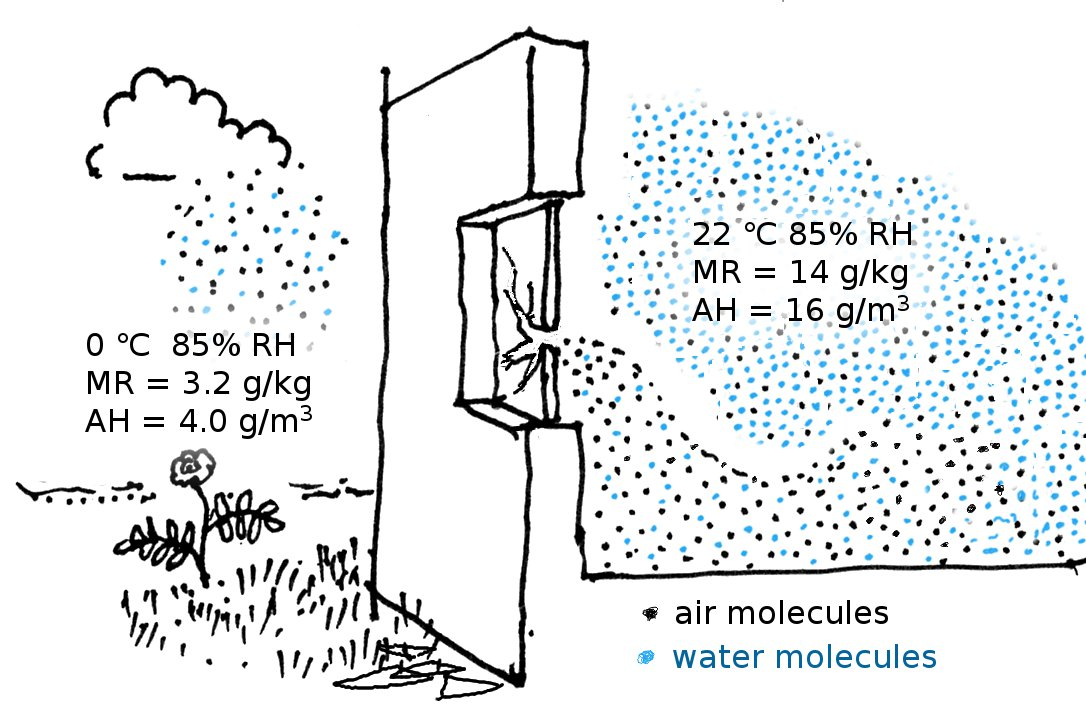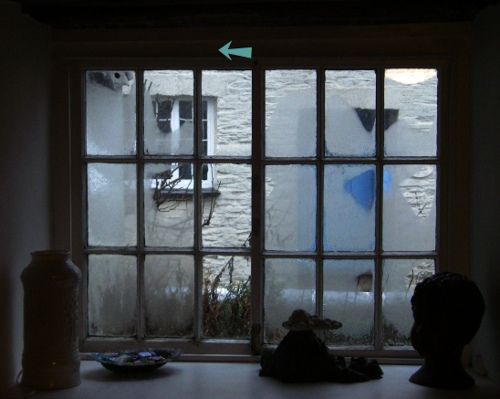The units used for describing water vapour in air are many, and confusing. This chapter is about Mixing Ratio (MR) and Absolute Humidity (AH).

The Mixing ratio (also called specific humidity) is a strange phrase but comes nearest to our intuitive understanding of concentration - the amount of one component in a mixture. The mixing ratio of water vapour in air is the weight of water vapour mixed into a given weight of dry air. The unit is kg/kg. This is the vertical axis of the psychrometric chart. There are two common uses for the mixing ratio. In meteorology the mixing ratio is useful for tracing the properties of vast air masses as they rise or fall in the atmosphere. The mixing ratio of a discrete blob of moist air does not change with varying atmospheric pressure and temperature as it moves in the atmosphere. In the more down to earth science of air conditioning, the mixing ratio is useful in calculations involving air as the transporter of water vapour through ducts to control the room humidity. Air is also the transporter of heat to control the room temperature. Calculations of the energy used in air conditioning have to combine the thermal properties of both air and water vapour.

In forensic studies of the microclimate in historic structures the mixing ratio is used to study the effects of infiltration, because its value is a much more sensitive way of differentiating between air streams than the relative humidity, which is the customary environmental measurement in museum conservation. On a cold day the outside air often has a high relative humidity but its mixing ratio is always quite small, because of the low saturation water vapour content at low temperature, and the relatively high density of cold air. At zero degrees and 85% RH the mixing ratio is just 0.0032 (kg/kg). Inside the steamy kitchen shown in the figure below, the RH is the same, but the temperature is higher. The air density is lower than outside but the water vapour is much more abundant, because the same RH at a higher temperature is represented by a higher water vapour concentration. The mixing ratio is 0.014. The kitchen has a broken window, so outside air is pouring in and flowing over the floor, because it is denser. Gradually it warms and mixes with the kitchen air as well as extracting moisture from absorbent furnishings. This process can be followed by watching the increase in mixing ratio as one traverses the room from the outside wall inwards.The mixing ratio requires knowing the total air pressure. In practice, the mixing ratio is calculated from measurement of the relative humidity and the temperature, assuming a typical air pressure of 101 kPa, at sea level.

Air moving into a room loses its identity rather quickly, as it mixes and warms. In the author's front room there is a horizontal sash window, which ensures a good air flow to the wood burning stove but also ensures a crisp chill in the room when the stove is unlit. On a cold winter morning most of the glass panes are fogged because they are the coldest surface in the room, so vapour from the furnishings and the ever-damp walls of the ancient house condenses there. However, the outside air, of equally high RH on this drizzly day, keeps the column of panes below the arrow well scrubbed with outside air flowing parallel to the glass through the vertical gap between the two halves of the window. These glass surfaces are actually cooler than the other panes, being exposed on both sides to outside air, However, there is no condensation because the stream of incoming air is above the dew point and quickly warms up as it enters the room, so the RH reduces further. The effect is very small scale - the panes of the leftmost column are just as fogged as those on the right side. The mixing ratio changes rapidly as the invading air merges with the room air.Use of the mixing ratio is therefore best confined to air masses so large that their water vapour exchange with the surroundings is very slow. Indoors, such isolation is only achieved within air conditioning ducts. In the free space of a room the mixing ratio quickly loses its identity. So I will next explain a near relative of the mixing ratio which is numerically very similar but gives a more direct insight into the vapour concentration in space.

The Absolute humidity of the air is the concentration of water vapour expressed as the weight of water in unit volume of space. In an isolated space this does change with pressure. because the space shrinks under pressure, pushing the water molecules closer together. However, in most situations relevant to conservation, except air transport and picture relining, the total atmospheric pressure remains nearly constant. The absolute humidity of a packet of air also changes slightly as it expands with temperature - proportionately to the kelvin temperature, meaning about one part in 300 per degree change in temperature.

### Analysis of the consequences of air exchange

Analysis of the effect of air infiltration on the relative humidity in a room begins with a measurement of the air exchange rate. This is usually calculated from the fall in concentration of a trace gas as it exits the room, at the room temperature. The rate is expressed as room volumes per hour. (An alternative technique is to slightly pressurise the room with a fan mounted in a temporary, close fitting door. One measures the air passing through the fan at a given pressure difference, then looks up the equivalent air exchange rate.)

If the outside air is colder, the air coming in will initially have a smaller volume than the air going out, because it will expand to match the outgoing volume as it warms up. During the warming process the mixing ratio does not change, so it can be directly compared with the mixing ratio of the room air as a first step towards calculating the change of room RH resulting from the mixing process.

If after one hour, the room has acquired 0.1 volumes of outside air, the mixing ratio is one tenth of the outside ratio plus nine tenths of the inside ratio. To find out if this matters to the room contents, the mixed up mixing ratio has to be converted to RH. Remembering that air has no influence on the RH, one first converts the mixing ratio to the absolute humidity in the interior space, which is then converted into the vapour pressure and finally into RH.

Here is an example calculation: at 20 °C the density of dry air is 1.2041 kg/m3. If the mixing ratio is 0.007 (kg/kg) the concentration (absolute humidity) of water vapour is 0.007 x 1.2041 = 0.0084 kg/m3. The vapour pressure is obtained from the gas law P = nRT. n is the mass of water vapour expressed in moles, which is 0.0084 divided by 0.018 kg which is the molar mass of water. This is = 0.47 mol. R is the gas constant: 8.31 Joules/mol/m3. This mysterious factor is just there to reconcile the units of the real participants. Remembering that T is in degrees kelvin, the partial pressure of water vapour is 0.47 x 8.31 x 293 = 1144 Pa. The saturation vapour pressure, taken from tables or formula, is 2326 Pa, so the RH is 1133/2326 = 49%.

Atmospheric water vapour is usually measured and recorded as RH, so to understand how RH changes with infiltration and other processes that mix two portions of air at different water content and temperature, one has to flip back and forth between four definitions for moisture in air: the mixing ratio, the absolute humidity, the vapour pressure and the relative humidity. However, in practice one can bypass the mixing ratio stage by calculating the absolute humidities of the air masses, if the temperatures are not vastly different. This is not strictly correct, but remember that all these quantities are in practice calculated from measurements of temperature, pressure, which is usually assumed, and relative humidity, which is difficult to measure with better than 2 % accuracy.

If you find all this rather complicated, there are even more esoteric formulae available in the recently published CEN standard 16242:2012 Procedures and instruments for measuring humidity in the air. This standard does not explain the derivation of the formulae, and it is a lot more expensive than this page.